MathScore EduFighter is one of the best math games on the Internet today. You can start playing for free!

Number Line - Sample Math Practice Problems

The math problems below can be generated by MathScore.com, a math practice program for schools and individual families. References to complexity and mode refer to the overall difficulty of the problems as they appear in the main program. In the main program, all problems are automatically graded and the difficulty adapts dynamically based on performance. Answers to these sample questions appear at the bottom of the page. This page does not grade your responses.

See some of our other supported math practice problems.

Complexity=2, Mode=1

Choose one or more answers that represent the point on the number line

 1.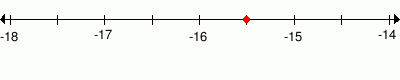-151/4        -151/2        -15.5        -16 2.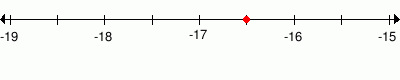-161/3        -17        -161/2        -16.5        -162/3

Complexity=4, Mode=1

Choose one or more answers that represent the point on the number line

 1.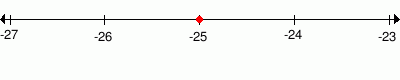-252/3        -25        -26        -251/2 2.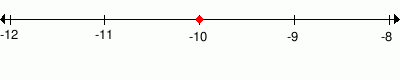-11        -10        -101/3        -101/2

Complexity=6, Mode=2

Choose one or more answers that represent the point on the number line

 1.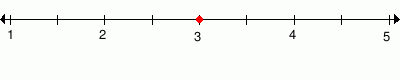3        31/3        4        31/2 2.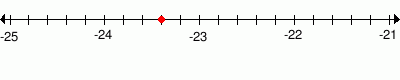-23.4        -235/7        -234/5        -232/5

Complexity=8, Mode=2

Choose one or more answers that represent the point on the number line

 1.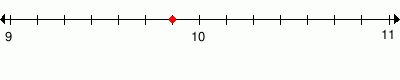96/7        91/3        91/7        93/8        10 2.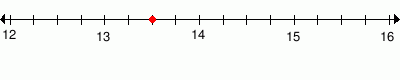14        131/3        132/3        13.5        131/2

Complexity=10, Mode=3

Choose one or more answers that represent the point on the number line

 1.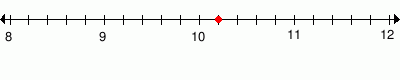10.2        101/5        11        101/7        102/5 2.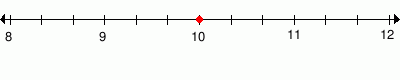10        46/1        101/2        11

Complexity=2, Mode=1

Choose one or more answers that represent the point on the number line

1-151/4        -151/2        -15.5        -16
Solution
-15 1/2
The whole number is -15 because the point is between -16 and -15
The numerator is 1 because the point is 1 notches to the left of -15.
The denominator is 2 because there are 2 equal parts between -16 and -15

-15.5 is the decimal value of -151/2
-15.5 = -15 + -5/10
= -151/2
2-161/3        -17        -161/2        -16.5        -162/3
Solution
-16 1/2
The whole number is -16 because the point is between -17 and -16
The numerator is 1 because the point is 1 notches to the left of -16.
The denominator is 2 because there are 2 equal parts between -17 and -16

-16.5 is the decimal value of -161/2
-16.5 = -16 + -5/10
= -161/2

Complexity=4, Mode=1

Choose one or more answers that represent the point on the number line

1-252/3        -25        -26        -251/2
Solution
-25
The whole number is -25 because the point is at -25.
2-11        -10        -101/3        -101/2
Solution
-10
The whole number is -10 because the point is at -10.

Complexity=6, Mode=2

Choose one or more answers that represent the point on the number line

13        31/3        4        31/2
Solution
3
The whole number is 3 because the point is at 3.
2-23.4        -235/7        -234/5        -232/5
Solution
-23 2/5
The whole number is -23 because the point is between -24 and -23
The numerator is 2 because the point is 2 notches to the left of -23.
The denominator is 5 because there are 5 equal parts between -24 and -23

-23.4 is the decimal value of -232/5
-23.4 = -23 + -4/10
= -232/5

Complexity=8, Mode=2

Choose one or more answers that represent the point on the number line

196/7        91/3        91/7        93/8        10
Solution
9 6/7
The whole number is 9 because the point is between 9 and 10
The numerator is 6 because the point is 6 notches to the right of 9.
The denominator is 7 because there are 7 equal parts between 9 and 10

214        131/3        132/3        13.5        131/2
Solution
13 2/4
The whole number is 13 because the point is between 13 and 14
The numerator is 2 because the point is 2 notches to the right of 13.
The denominator is 4 because there are 4 equal parts between 13 and 14

131/2 is a simplified fraction of 13 2/4

13.5 is the decimal value of 131/2
13.5 = 13 + 5/10
= 131/2

Complexity=10, Mode=3

Choose one or more answers that represent the point on the number line

110.2        101/5        11        101/7        102/5
Solution
10 1/5
The whole number is 10 because the point is between 10 and 11
The numerator is 1 because the point is 1 notches to the right of 10.
The denominator is 5 because there are 5 equal parts between 10 and 11

10.2 is the decimal value of 101/5
10.2 = 10 + 2/10
= 101/5
210        46/1        101/2        11
Solution
10
The whole number is 10 because the point is at 10.
46/1 simplifies to 10
46/1 = 4 + 6/1 = 4 + 6
= 10